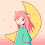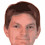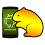## Sunday, 14 November 2010

### RGB/HSV in HLSL

My present work has brought me into contact with HLSL pixel shaders. Many of these involve colour manipulations, so a fast and efficient conversions between RGB and HSV (or HSL) colour spaces would seem to be readily available and optimised to death. Strangely, this doesn't seem to be the case. Even shaders given as examples by NVIDIA and ATI are somewhat simplistic. The Wikipedia page's pseudo-code suggested the following HLSL code:

float3 HSVtoRGB(float3 HSV)
{
float3 RGB = 0;
float C = HSV.z * HSV.y;
float H = HSV.x * 6;
float X = C * (1 - abs(fmod(H, 2) - 1));
if (HSV.y != 0)
{
float I = floor(H);
if (I == 0) { RGB = float3(C, X, 0); }
else if (I == 1) { RGB = float3(X, C, 0); }
else if (I == 2) { RGB = float3(0, C, X); }
else if (I == 3) { RGB = float3(0, X, C); }
else if (I == 4) { RGB = float3(X, 0, C); }
else { RGB = float3(C, 0, X); }
}
float M = HSV.z - C;
return RGB + M;
}

float3 RGBtoHSV(float3 RGB)
{
float3 HSV = 0;
float M = min(RGB.r, min(RGB.g, RGB.b));
HSV.z = max(RGB.r, max(RGB.g, RGB.b));
float C = HSV.z - M;
if (C != 0)
{
HSV.y = C / HSV.z;
float3 D = (((HSV.z - RGB) / 6) + (C / 2)) / C;
if (RGB.r == HSV.z)
HSV.x = D.b - D.g;
else if (RGB.g == HSV.z)
HSV.x = (1.0/3.0) + D.r - D.b;
else if (RGB.b == HSV.z)
HSV.x = (2.0/3.0) + D.g - D.r;
if ( HSV.x < 0.0 ) { HSV.x += 1.0; }
if ( HSV.x > 1.0 ) { HSV.x -= 1.0; }
}
return HSV;
}

Even to my eyes, these looked far than optimal. However, a quick glance at the HSV graph on the Wiki page suggests an optimisation for 'HSVtoRGB' which doesn't involve branching or conditional predicates.

float3 Hue(float H)
{
float R = abs(H * 6 - 3) - 1;
float G = 2 - abs(H * 6 - 2);
float B = 2 - abs(H * 6 - 4);
return saturate(float3(R,G,B));
}

float3 HSVtoRGB(in float3 HSV)
{
return ((Hue(HSV.x) - 1) * HSV.y + 1) * HSV.z;
}

This is particularly efficient because 'abs' and 'saturate' are "free" operations on a lot of GPU pipelines.

The reverse conversion is a bit more tricky, and if you're really obsessed with performance on Xbox360, or the like, you'll need to drop down into shader assembler to utilise the vector 'max4' instruction instead of the scalar alternatives:

float3 RGBtoHSV(in float3 RGB)
{
float3 HSV = 0;
#if NO_ASM
HSV.z = max(RGB.r, max(RGB.g, RGB.b));
float M = min(RGB.r, min(RGB.g, RGB.b));
float C = HSV.z - M;
#else
float4 RGBM = RGB.rgbr;
asm { max4 HSV.z, RGBM };
asm { max4 RGBM.w, -RGBM };
float C = HSV.z + RGBM.w;
#endif
if (C != 0)
{
HSV.y = C / HSV.z;
float3 Delta = (HSV.z - RGB) / C;
Delta.rgb -= Delta.brg;
Delta.rg += float2(2,4);
if (RGB.r >= HSV.z)
HSV.x = Delta.b;
else if (RGB.g >= HSV.z)
HSV.x = Delta.r;
else
HSV.x = Delta.g;
HSV.x = frac(HSV.x / 6);
}
return HSV;
}

Although we haven't managed to get rid of the 'if' statements, they typically compile down to one conditionally predicated block and two conditional assignments.

Even against the startlingly successful optimisations produced by the current batch of HLSL compilers, these refactorings produce excellent results. The round-trip conversions (RGB-to-HSV-to-RGB) are typically three times faster than the simplistic implementations. For a pixel shader, that's not to be sneezed at.

1.Not sure if it's specific to my setup, but I had to modify the RGBtoHSV function for my use as I found that the floating-point comparison tests were producing weird behavior for me. I think it has to do with edge cases where RGB.r or RGB.g is supposed to be equal to HSV.z. For example, I have a yellow RGB color that turns blue if I convert to HSV and then back to RGB, but if I use the following:

if (RGB.r+0.1 >= HSV.z)
HSV.x = Delta.b;
else if (RGB.g+0.1 >= HSV.z)
HSV.x = Delta.r;
else
HSV.x = Delta.g;

it remains yellow.

here's "a version which works". Don't mind the braindead sloppiness; i'm just trying to illustrate the problem.

float3 RGBtoHSV(in float3 RGB)
{
int whichmax = 0;
if (RGB.g > RGB.r)
{
if (RGB.b > RGB.g)
whichmax = 2;
else
whichmax = 1;
}
else if (RGB.b > RGB.r)
whichmax = 2;

float3 HSV = 0;
HSV.z = max(RGB.r, max(RGB.g, RGB.b));
float M = min(RGB.r, min(RGB.g, RGB.b));
float C = HSV.z - M;
if (C != 0)
{
HSV.y = C / HSV.z;
float3 Delta = (HSV.z - RGB) / C;
Delta.rgb -= Delta.brg;
Delta.rg += float2(2,4);
if (whichmax == 0)
HSV.x = Delta.b;
else if (whichmax == 1)
HSV.x = Delta.r;
else
HSV.x = Delta.g;
HSV.x = frac(HSV.x / 6);
}

return HSV;
}

2.Hmmm, DDRKirby(ISQ), that's very strange. I've checked the HSV-to-RGB and RGB-to-HSV results against three other implementations without significant disagreements. I've also checked the round-trip results to make sure that HSV-to-RGB-to-HSV and RGB-to-HSV-to-RGB are sensible. I don't see any anomalies there, either. What GPU are you running on?

[Sorry for the delay in getting back to you, but I've been testing this as extensively as I can in my spare time over the holidays]

3.Hi Ian,

Thank you very much for your code. I rewrote your formula using the SSE intrinsics and it works perfectly. It will be available in Fog.

Now I'd like to hear that the second formula can be also optimized using min/max/abs, but it's not trivial:)

Thank you

4.Petr,

Your comments on SSE nudged a corner of my brain. I've had a look at this over the last couple of days and think there might be some room for improvement, but it requires what SIMD geeks apparently call "horizontal minimum for packed floats" (see http://software.intel.com/en-us/forums/showthread.php?t=79647).

The whole "if" statement in the "RGBtoHSV" code above can be replaced with the following:

float D = step(0, -C);
HSV.y = C / (HSV.z + D);
float3 Delta = (HSV.z - RGB) / (C + D);
Delta -= Delta.brg;
Delta += float3(2,4,6);
Delta *= step(HSV.zzz, RGB.gbr);
#if NO_ASM
HSV.x = max(Delta.r, max(Delta.g, Delta.b));
#else
float4 Delta4 = Delta.rgbr;
asm { max4 HSV.x, Delta4 };
#endif
HSV.x = frac(HSV.x / 6);

I'll check whether this improves typical pixel shader performance (I have doubts, because of the way predication works) but it might be an fruitful avenue to pursue in SSE land.

5.Hi Ian,

it's betting better and better :) I have also some inventions, but I don't understand the

float D = step(0, -C);

in your formula. Isn't the result always 1? (C is between 0 and 1 and -0 to -1 is always <= 0, true?)

But I'm getting some ideas how to avoid efficiently division by zero. I need also to avoid frac().

6.In HLSL, "step(y,x)" is equivalent to "(x>=y)?1:0". As you correctly point out, C is between zero and one inclusive, so the substituted expression "(-C>=0)?1:0" is simply "(C==0)?1:0". A bit ugly, but does the job.

In SSE land, could you use "CMPEQPS" or the like?

7.Hi Ian,

sorry about the step(y, x), I missed the reversed order of arguments, I read x, y in MSDN :) I have no experience with shaders so these functions are new to me.

In SSE it's possible to use lower-level tricks with masks, I will send you my version when ready.

8.BTW: Yes, CMPEQPS/CMPEQSS are candidates

9.Hi, i've been looking for fast hsl/hsv/rgb transforms and found your blog, thanks for sharing.

I am trying to implement photoshop color blending that replaces both hue and saturation of the image' pixel but preserves lightness. I am doing it under android os using gl es 2.0 and fragment shaders.

I creates texture (render) buffer to store HSL data of the original texture. Then i passes it to pixel shader, applies hsl modifications and transforms back to rgb values.

I am using your code to convert rgb->hsl and vise versa and it works perfect. Everything works correct but it seems like there is not enough presicion for lighting/saturation to store in regular unsigned byte rgba texture.

In order to preserve precision i am using the following conversion:

float hue = hsl.x * 1024.0;
float b1 = floor(hue / 32.0);
float b2 = hue - b1 * 32.0;

gl_FragColor = vec4(b1 / 32.0, b2 / 32.0, hsl.y, hsl.z);

Is means R and G components are used to store hue value (0 to 360 that is greater than max value of 255 of unsigned byte) with 2^-10 precision (most likely supported precision on android devices).

Than i unpacks it back in another fragment shader

vec4 src = texture2D(texHSV, uv);
vec3 hsv = vec3((32.0 * src.r + src.g) / 32.0, src.b, src.a);

When i used this packing/unpacking to store HSV values everything works correct. But when switching to HSL i got sharp differences on S and L near 1.0.

May be you can give me some advice or something?) I wasted hours to get it worked, but currently have no mode ideas...

10.Oh, i just got that my problem is not the precision i telling before.

Color blend mode that i want to implement is similar to photoshop's color blend that is not HSL in terms of lighting. L stands for luma also known as Y and i need to convert RGB -> HSY and vise versa.

But currently can't find fast alhorithm to do HSY -> RGB conversion.

11.Hi Hamster Beat, I've had a quick look at your comments. As you say, I don't think it's a precision problem; most modern pipelines use floating point internals, so scaling uniformly by constants rarely affects precision.

If, as you say (and Wikipedia suggests) the HSY model expects the Y component to be luma (weighted brightness according to some physical absorption model, e.g. the human eye) then RGB->HSY would simply require the R G and B multiplicative weights to be added to the formula. HSY->RGB should follow on from that, although you'd have to be sareful to make it reversible. I'll have a look at it during the week and see what I can come up with.

12.HCY seems to be a rarely-used colour space, which gives me pause for thought. But I did find references to it in this Google Code project:

I'll try to unpick the code.

13.P.S. I'm assuming you really want code for "HCY"; "HSY" seems to be a bit of a Franken-colour space.

14.Another fast (possibly faster - I have yet to benchmark it) RGB to HSV algorithm is : http://lolengine.net/blog/2013/07/27/rgb-to-hsv-in-glsl

1.Yes. That's a rather sweet optimization for RGB-to-HSV. It doesn't seem to have an impact on modern scalar GPUs, but definitely faster for the SIMD generation. I'll write up an updated entry when I can. Sam's HSV-to-RGB is a little slower, so maybe we can trade ;-)